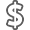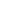# A First Course in Probability

• ISBN13:

• ISBN10:

## 0134753119

• Edition: 10th
• Format: Hardcover
• Publisher: Pearson

Note: Supplemental materials are not guaranteed with Rental or Used book purchases.

Purchase Benefits

•Free Shipping On Orders Over \$35!
Your order must be \$35 or more to qualify for free economy shipping. Bulk sales, PO's, Marketplace items, eBooks and apparel do not qualify for this offer.
•Get Rewarded for Ordering Your Textbooks! Enroll Now
•We Buy This Book Back!
In-Store Credit: \$77.44
Check/Direct Deposit: \$73.75
PayPal: \$73.75
List Price: \$226.64 Save up to \$177.15
• Rent Book \$79.32Free Shipping

TERM
PRICE
DUE
IN STOCK USUALLY SHIPS WITHIN 24-48 HOURS.
*This item is part of an exclusive publisher rental program and requires an additional convenience fee. This fee will be reflected in the shopping cart.

### Supplemental Materials

What is included with this book?

• The New copy of this book will include any supplemental materials advertised. Please check the title of the book to determine if it should include any access cards, study guides, lab manuals, CDs, etc.
• The Used, Rental and eBook copies of this book are not guaranteed to include any supplemental materials. Typically, only the book itself is included. This is true even if the title states it includes any access cards, study guides, lab manuals, CDs, etc.

### Summary

For upper-level to graduate courses in Probability or Probability and Statistics, for majors in mathematics, statistics, engineering, and the sciences.

Explores both the mathematics and the many potential applications of probability theory

A First Course in Probability is an elementary introduction to the theory of probability for students in mathematics, statistics, engineering, and the sciences. Through clear and intuitive explanations, it presents not only the mathematics of probability theory, but also the many diverse possible applications of this subject through numerous examples. The 10th Edition includes many new and updated problems, exercises, and text material chosen both for interest level and for use in building student intuition about probability.

0134753119 / 9780134753119 A First Course in Probability, 10/e

### Author Biography

Sheldon M. Ross is a professor in the Department of Industrial Engineering and Operations Research at the University of Southern California. He received his Ph.D. in statistics at Stanford University in 1968. He has published many technical articles and textbooks in the areas of statistics and applied probability. Among his texts are A First Course in Probability, Introduction to Probability Models, Stochastic Processes, and Introductory Statistics. Professor Ross is the founding and continuing editor of the journal Probability in the Engineering and Informational Sciences, the Advisory Editor for International Journal of Quality Technology and Quantitative Management, and an Editorial Board Member of the Journal of Bond Trading and Management.  He is a Fellow of the Institute of Mathematical Statistics and a recipient of the Humboldt US Senior Scientist Award.

1. COMBINATORIAL ANALYSIS

1.1 Introduction

1.2 The Basic Principle of Counting

1.3 Permutations

1.4 Combinations

1.5 Multinomial Coefficients

1.6 The Number of Integer Solutions of Equations

Summary

Problems

Theoretical Exercises

Self-Test Problems and Exercises

2. AXIOMS OF PROBABILITY

2.1 Introduction

2.2 Sample Space and Events

2.3 Axioms of Probability

2.4 Some Simple Propositions

2.5 Sample Spaces Having Equally Likely Outcomes

2.6 Probability as a Continuous Set Function

2.7 Probability as a Measure of Belief

Summary

Problems

Theoretical Exercises

Self-Test Problems and Exercises

3. CONDITIONAL PROBABILITY AND INDEPENDENCE

3.1 Introduction

3.2 Conditional Probabilities

3.3 Bayes’s Formula

3.4 Independent Events

3.5 PF) Is a Probability

Summary

Problems

Theoretical Exercises

Self-Test Problems and Exercises

4. RANDOM VARIABLES

4.1 Random Variables

4.2 Discrete Random Variables

4.3 Expected Value

4.4 Expectation of a Function of a Random Variable

4.5 Variance

4.6 The Bernoulli and Binomial Random Variables

4.7 The Poisson Random Variable

4.8 Other Discrete Probability Distributions

4.9 Expected Value of Sums of Random Variables

4.10 Properties of the Cumulative Distribution Function

Summary

Problems

Theoretical Exercises

Self-Test Problems and Exercises

5. CONTINUOUS RANDOM VARIABLES

5.1 Introduction

5.2 Expectation and Variance of Continuous Random Variables

5.3 The Uniform Random Variable

5.4 Normal Random Variables

5.5 Exponential Random Variables

5.6 Other Continuous Distributions

5.7 The Distribution of a Function of a Random Variable

Summary

Problems

Theoretical Exercises

Self-Test Problems and Exercises

6. JOINTLY DISTRIBUTED RANDOM VARIABLES

6.1 Joint Distribution Functions

6.2 Independent Random Variables

6.3 Sums of Independent Random Variables

6.4 Conditional Distributions: Discrete Case

6.5 Conditional Distributions: Continuous Case

6.6 Order Statistics

6.7 Joint Probability Distribution of Functions of Random Variables

6.8 Exchangeable Random Variables

Summary

Problems

Theoretical Exercises

Self-Test Problems and Exercises

7. PROPERTIES OF EXPECTATION

7.1 Introduction

7.2 Expectation of Sums of Random Variables

7.3 Moments of the Number of Events that Occur

7.4 Covariance, Variance of Sums, and Correlations

7.5 Conditional Expectation

7.6 Conditional Expectation and Prediction

7.7 Moment Generating Functions

7.8 Additional Properties of Normal Random Variables

7.9 General Definition of Expectation

Summary

Problems

Theoretical Exercises

Self-Test Problems and Exercises

8. LIMIT THEOREMS 394

8.1 Introduction

8.2 Chebyshev’s Inequality and the Weak Law of Large Numbers

8.3 The Central Limit Theorem

8.4 The Strong Law of Large Numbers

8.5 Other Inequalities and a Poisson Limit Result

8.6 Bounding the Error Probability When Approximating a Sum of Independent Bernoulli Random Variables by a Poisson Random Variable

8.7 The Lorenz Curve

Summary

Problems

Theoretical Exercises

Self-Test Problems and Exercises

9.1 The Poisson Process

9.2 Markov Chains

9.3 Surprise, Uncertainty, and Entropy

9.4 Coding Theory and Entropy

Summary

Problems and Theoretical Exercises

Self-Test Problems and Exercises

10. SIMULATION

10.1 Introduction

10.2 General Techniques for Simulating Continuous Random Variables

10.3 Simulating from Discrete Distributions

10.4 Variance Reduction Techniques

Summary

Problems

Self-Test Problems and Exercises

Solutions to Self-Test Problems and Exercises

Index

Common Discrete Distributions

Common Continuous Distributions

### Supplemental Materials

What is included with this book?

The New copy of this book will include any supplemental materials advertised. Please check the title of the book to determine if it should include any access cards, study guides, lab manuals, CDs, etc.

The Used, Rental and eBook copies of this book are not guaranteed to include any supplemental materials. Typically, only the book itself is included. This is true even if the title states it includes any access cards, study guides, lab manuals, CDs, etc.